### Home > PC > Chapter 2 > Lesson 2.3.5 > Problem2-127

2-127.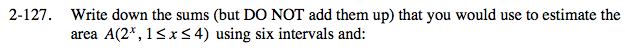Find the width.

$\text{width }=\frac{\text{interval}}{\text{no. of pieces}}$

Draw the graph and label.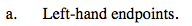left − hand approximation = 0.5(21 +21.5 + 22 + 22.5 + 23.5)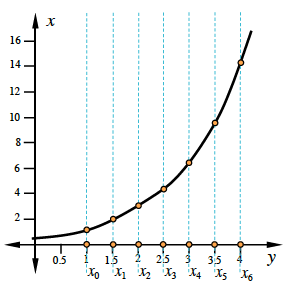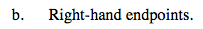Be careful with the start and end heights!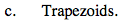Draw all of the trapezoids. Then calculate the area for each.

$=\text{width}\cdot \frac{(\text{base}_{1}+\text{base}_{2})}{2}\text{ for each trapezoid}$